## How to Calculate and Solve for Centripetal Acceleration, Angular Velocity and Radius of Circular Path of a Body | The Calculator Encyclopedia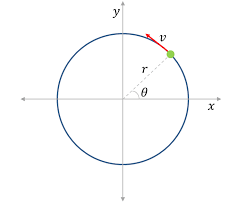The image above represents centripetal acceleration in a motion of circular path.

To compute for the centripetal acceleration, two essential parameters are needed and these parameters are angular velocity (ω) and radius of circular path (r).

The formula for the calculating centripetal acceleration:

a = ω²r

Where:
a = Centripetal Acceleration
ω = Angular Velocity
r = Radius of Circular Path

Let’s solve an example;
Find the centripetal acceleration with an angular velocity of 33 and a radius of 21.

This implies that;
ω = Angular Velocity = 33
r = Radius of Circular Path = 21

a = ω²r
a = 33² x 21
a = 1089 x 21
a = 22869

Therefore, the centripetal acceleration is 22869 m/s².

Calculating the Angular Velocity when Centripetal Acceleration and Radius of Circular path is Given.

ω = √a / r

Where:
ω = Angular Velocity
a = Centripetal Acceleration
r = Radius of Circular Path

Let’s solve an example;
Find the angular velocity with a centripetal acceleration of 320 and a radius of 8.

This implies that;
a = Centripetal Acceleration = 320
r = Radius of Circular Path = 8

ω = √a / r
ω = √320 / 8
ω = √40
ω = 6.32

Therefore, the angular velocity is 6.32.

## How to Calculate and Solve for Maximum Velocity, Coefficient of Friction and Radius of a Body in Motion of Circular Path | Nickzom Calculator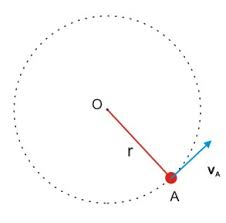The image above represents maximum velocity in circular motion.

To compute for the maximum velocity, three essential parameters are needed and these parameters are coefficient of friction (μ), radius (r) and acceleration due to gravity (g).

The formula for calculating maximum velocity:

Vmax = √(μgr)

Where;
Vmax = maximum velocity
μ = coefficient of friction
g = acceleration due to gravity

Let’s solve an example;
Find the maximum velocity when the coefficient of friction is 14 with a radius of 7 and acceleration due to gravity of 9.8.

This implies that;
μ = coefficient of friction = 14
g = acceleration due to gravity = 9.8

Vmax = √(μgr)
Vmax = √(14 x 7 x 9.8)
Vmax = √(960.40)
Vmax = 30.99

Therefore, the maximum velocity is 30.99 m/s.

Calculating the Coefficient of Friction when the Maximum Velocity, Radius and Acceleration due to Gravity is Given.

μ = Vmax2 / gr

Where;
μ = coefficient of friction
Vmax = maximum velocity
g = acceleration due to gravity

Let’s solve an example;
Find the coefficient of friction with a maximum velocity of 120, radius of 15 and acceleration due to gravity is 9.8?

This implies that;
Vmax = maximum velocity = 120
g = acceleration due to gravity = 9.8

μ = Vmax2 / gr
μ = 1202 / 15 x 9.8
μ = 14400 / 147
μ = 97.96

Therefore, the coefficient of friction is 97.96.

## How to Calculate and Solve for Superelevation, Guage of Track, Velocity and Radius of a Body in Circular Path Motion | The Calculator Encyclopedia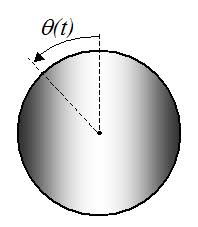The image above represents a body in superelevation.

To compute for the Superelevation, four essential parameters are needed and these parameters are Gauge of the track (G), velocity of the body (v), radius of the curve (r) and acceleration due to gravity (g).

The formula for calculating the circular path motion:

S = Gv² / gr

Where:
S = Superelevation
G = Gauge of the track
v = velocity of the body
r = radius of the curve
g = acceleration due to gravity

Let’s solve an example;
Find the circular path motion when Gauge of the track is 14, velocity of the track is 47, radius of the curve is 21 and acceleration due to gravity is 9.8.

This implies that;
G = Gauge of the track = 14
v = velocity of the body = 47
r = radius of the curve = 21
g = acceleration due to gravity = 9.8

S = Gv² / gr
S = 14 x 47² / 9.8 x 21
S = 14 x 2209 / 205.8
S = 30926 / 205.8
S = 150.27

Therefore, the superelevation is 150.27 m.

Calculating the Gauge of the track when Superelevation, Velocity of the body, Radius of the curve and Acceleration due to gravity.

G = Sgr / v2

Where;
G = Gauge of the track
S = Superelevation
v = velocity of the body
r = radius of the curve
g = acceleration due to gravity

Let’s solve an example;
With a superelevation of 180, velocity of the body is 32, radius of the curve is 12 and acceleration due to gravity as 9.8. Find the gauge of the track?

This implies that;
S = Superelevation = 180
v = velocity of the body = 32
r = radius of the curve = 12
g = acceleration due to gravity = 9.8

G = Sgr / v2
G = 180 x 12 x 9.8 / 322
G = 21168 / 1024
G = 20.67

Therefore, the gauge of the track is 20.67.

Calculating the Velocity of the body when Superelevation, Gauge of the track, Radius of the curve and Acceleration due to gravity.

v = √Sgr / G

Where;
v = velocity of the body
S = Superelevation
G = Gauge of the track
r = radius of the curve
g = acceleration due to gravity

Let’s solve an example;
With a superelevation of 120, gauge of the track is 28, radius of the curve is 7 and acceleration due to gravity as 9.8. Find the velocity of the body?

This implies that;
S = Superelevation = 120
G = gauge of the track = 28
r = radius of the curve = 7
g = acceleration due to gravity = 9.8

v = √Sgr / G
v = √120 x 9.8 x 7 / 28
v = √8232 / 28
v = √294
v = 17.146

Therefore, the velocity of the body is 17.146.

## How to Calculate and Solve for Number of Revolutions per Minute and Angular Velocity of Motion of Circular Path | The Calculator Encyclopedia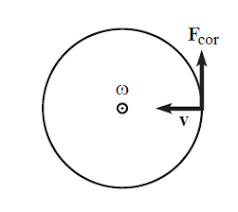The image above represent angular velocity.

To compute the angular velocity, one essential parameter is needed and its parameter is Number of Revolutions per Minute (N).

The formula for calculating angular velocity:

ω = 2πN / 60

Where;
ω = Angular velocity
N = Number of revolutions per minute

Let’s solve an example;
Find the Angular Velocity with a number of revolutions per minute as 60.

This implies that;
N = Number of revolutions per minute = 60

ω = 2πN / 60
ω = 2 x π x 24 / 60
ω = 150.816/ 60
ω = 2.5136

Therefore, the angular velocity is 2.5136 rad/s.

Calculating the Number of Revolutions per Minute when Angular Velocity is Given.

N = ω60 /

Where;
N = Number of revolutions per minute
ω = Angular velocity

Let’s solve an example;
With an angular velocity of 40. Find the number of revolutions per minute?

This implies that;
ω = Angular velocity = 40

N = ω60 /
N = 40 x 60 / 6.284
N = 2400 / 6.284
N = 381.9

Therefore, the number of revolutions per minute is 381.9 min.

## How to Calculate and Solve for Mass, Angular Velocity, Radius and Centrifugal Force of a Body | The Calculator Encyclopedia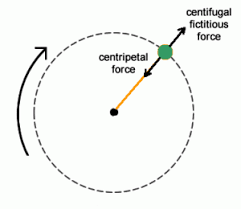The image above represents the centrifugal force.

To compute for the centrifugal force, three essential parameters are needed and these parameters are Mass of the body (m), Angular Velocity of the body (w) and Radius (r).

The formula for calculating the centrifugal force:

F = mω²r

Where:
F = Centrifugal Force
m = mass of the body
ω = angular velocity

Let’s solve an example;
Find the centrifugal force with mass of the body as 12, angular velocity as 32 and a radius of 8.

This implies that;
m = mass of the body = 12
ω = angular velocity = 32

F = mω²r
F = 12 x 32² x 8
F = 12 x 1024 x 8
F = 98304

Therefore, the centrifugal force is 98304 N.

Calculating the Mass of the body (m) when the Centrifugal Force, Angular Velocity and Radius is Given.

m = F / w2r

Where;
m = mass of the body
F = Centrifugal Force
ω = angular velocity

Let’s solve an example;
Find the mass of a body when centrifugal force is 140 with an angular velocity of 24 and a radius of 10.

This implies that;
F = Centrifugal Force = 140
ω = angular velocity = 24

m = F / w2r
m = 140 / 24210
m = 140 / 576 x 10
m = 140 / 5760
m = 0.024

Therefore, the mass of the body is 0.024 kg.

## How to Calculate and Solve for Mass, Height and Potential Energy | The Calculator Encyclopedia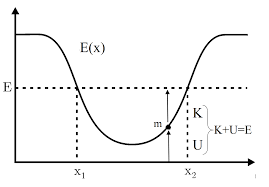The image above represents potential energy.

To compute for the potential energy, three essential parameters are needed and these parameters are mass (m), height (h) and acceleration due to gravity (g).

The formula for calculating the potential energy:

P.E = mgh

Where;
P.E. = Potential Energy
m = Mass
g = acceleration due to gravity
h = Height

Let’s solve an example;
Find the potential energy when the mass is 12 with a height of 24 and acceleration due to gravity of 9.8.

This implies that;
m = Mass = 12
g = acceleration due to gravity = 9.8
h = Height = 24

P.E = mgh
P.E = 12 x 9.8 x 24
P.E = 2822.4

Therefore, the potential energy is 2822.4 Joules (J).

Calculating the Mass when Potential Energy, Height and Acceleration due to Gravity is Given.

m = P.E / gh

Where;
m = Mass
P.E. = Potential Energy
g = acceleration due to gravity
h = Height

Let’s solve an example;
Find the Mass when potential energy is 450 with a height of 30 and acceleration due to gravity of 10.

This implies that;
P.E. = Potential Energy = 450
g = acceleration due to gravity = 10
h = Height = 30

m = P.E / gh
m = 450 / 10 x 30
m = 450 / 300
m = 1.5

Therefore, the mass is 1.5.

## How to Calculate and Solve for Mass, Velocity and Kinetic Energy | The Calculator Encyclopedia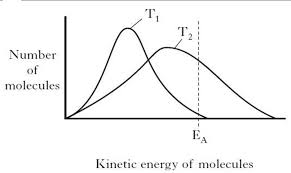The image above represents kinetic energy.

To compute for the kinetic energy, two essential parameters are needed and these parameters are mass (m) and velocity (v).

The formula for calculating the kinetic energy:

K.E. = 0.5mv²

Where;
K.E. = Kinetic Energy
m = Mass
v = Velocity

Let’s solve an example;
Find the kinetic energy when the mass is 6 and the velocity is 18.

This implies that;
m = Mass = 6
v = Velocity = 18

K.E. = 0.5mv²
K.E. = 0.5[6 x 18²]
K.E. = 0.5[6 x 324]
K.E. = 0.5
K.E. = 972

Therefore, the kinetic energy is 972 Joules (J).

Calculating the Mass when Kinetic Energy and Velocity is Given.

m = K.E / 0.5v2

Where;
m = Mass
K.E. = Kinetic Energy
v = Velocity

Let’s solve an example;
Find the mass when the kinetic energy is 320 and a velocity of 20.

This implies that;
K.E. = Kinetic Energy = 320
v = Velocity = 20

m = K.E / 0.5v2
m = 320 / 0.5 x 202
m = 320 / 0.5 x 40
m = 320 / 20
m = 16

Therefore, the mass is 16 kg.

## How to Calculate and Solve for Mass, Volume and Linear Momentum | The Calculator Encyclopedia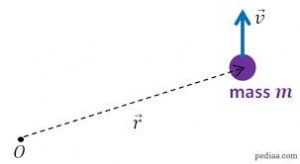The image above represents a linear momentum.

To compute for the linear momentum, two essential parameters are needed and these parameters are mass (m) and velocity (v).

The formula for calculating linear momentum:

p = mv

Where;
p = Momentum
m = Mass
v = Velocity

Let’s solve an example;
Find the linear momentum of a mass of 44 and a velocity of 38.

This implies that;
m = Mass = 44
v = Velocity = 38

p = mv
p = 44 x 38
p = 1672

Therefore, the linear momentum is 1672 Kgm/s.

Calculating the Mass when Linear Momentum and Velocity is Given.

m = p / v

Where;
m = Mass
p = Momentum
v = Velocity

Let’s solve an example;
Find the mass with a linear momentum of 320 and a velocity of 80.

This implies that;
p = Momentum = 320
v = Velocity = 80

m = p / v
m = 320 / 80
m = 4

Therefore, the mass is 4 kg.

## How to Calculate and Solve for the Quantity of Charge, Electrochemical Equivalence of a Substance and Mass of an Element in Electrolysis | Nickzom Calculator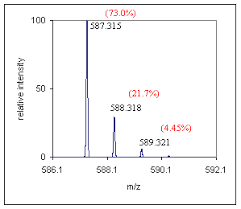The image above represents the mass of an element.

To compute for the mass of an element, two essential parameters are needed and these parameters are Electrochemical Equivalence of the Substance (Z) and quantity of charge (Q).

The formula for calculating mass of an element:

M = ZQ

Where;
M = Mass of the element
Z = Electrochemical Equivalence of the Substance
Q = Quantity of Charge

Let’s solve an example;
Find the mass of an element when the Quantity of charge is 28 and Electrochemical Equivalence of the Substance is 32.

This implies that;
Z = Electrochemical Equivalence of the Substance = 32
Q = Quantity of Charge = 28

M = ZQ
M = 32 x 28
M = 896

Therefore, the mass of an element is 896 kg.

Calculating the Electrochemical Equivalence of the Substance when the Mass of an Element and Quantity of Charge is Given.

Z = M / Q

Where;
Z = Electrochemical Equivalence of the Substance
M = Mass of the element
Q = Quantity of Charge

Let’s solve an example;
Find the Electrochemical Equivalence of the Substance when the Quantity of charge is 12 and  mass of an element  is 120.

This implies that;
M = Mass of the element = 120
Q = Quantity of Charge = 12

Z = M / Q
Z = 120 / 12
Z = 10

Therefore, the Electrochemical Equivalence of the Substance is 10.

## How to Calculate and Solve for the Current, Time and Quantity of Charge of an Electrolysis | The Calculator Encyclopedia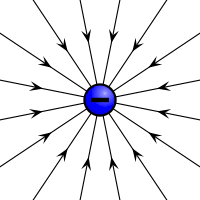The image above represents the quantity of charge.

To compute for the quantity of charge, two essential parameters are needed and these parameters are current (I) and time (T).

The formula for calculating the quantity of charge:

Q = It

Where;
Q = Quantity of charge
I = Current
T = Time

Let’s solve an example;
Find the quantity of charge with a current of 24 and time of 12.

This implies that;
I = Current = 24
T = Time = 12

Q = It
Q = 24 x 12
Q = 288

Therefore, the quantity of charge is 288 Coulombs (C).

Calculating the Current (I) using the Quantity of Charge and Time.

I = Q / t

Where;
I = Current
Q = Quantity of charge
T = Time

Let’s solve an example;
Given that the quantity of charge is 240 with a time of 14. Find the Current?

This implies that;
Q = Quantity of charge = 240
T = Time = 14

I = Q / t
I = 240 / 14
I = 17.14

Therefore, the current is 17.14 ampere.